# How to Calculate and Solve for Heat Flux | Solidification of Metals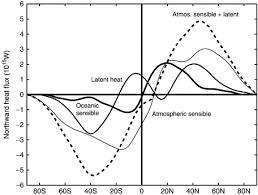The image above represents heat flux.

To compute for heat flux, three essential parameters are needed and these parameters are Heat Emission (h), Surface Temperature (Ts) and Initial Temperature (To).

The formula for calculating heat flux:

qx = h(Ts – To)

Where:

qx = Heat Flux (T)
h = Heat Emission
Ts = Surface Temperature
To = Initial Temperature

Let’s solve an example;
Find the heat flux when the heat emission is 21, the surface temperature is 12 and the initial temperature is 10.

This implies that;

h = Heat Emission = 21
Ts = Surface Temperature = 12
To = Initial Temperature = 10

qx = h(Ts – To)
qx = 21(12 – 10)
qx = 21(2)
qx = 42

Therefore, the heat flux is 42 W.

Calculating the Heat Emission when the Heat Flux, the Surface Temperature and the Initial Temperature is Given.

h = qx / Ts – To

Where:

h = Heat Emission
qx = Heat Flux (T)
Ts = Surface Temperature
To = Initial Temperature

Let’s solve an example;
Find the heat emission when the heat flux is 40, the surface temperature is 20 and the initial temperature is 10.

This implies that;

qx = Heat Flux (T) = 40
Ts = Surface Temperature = 20
To = Initial Temperature = 10

h = qx / Ts – To
h = 40 / 20 – 10
h = 40 / 10
h = 4

Therefore, the heat emission is 4.

Calculating the Surface Temperature when the Heat Flux, the Heat Emission and the Initial Temperature is Given.

Ts = (qx / h) + To

Where:

Ts = Surface Temperature
qx = Heat Flux (T)
h = Heat Emission
To = Initial Temperature

Let’s solve an example;
Find the surface temperature when the heat flux is 24, the heat emission is 6 and the initial temperature is 4.

This implies that;

qx = Heat Flux = 24
h = Heat Emission = 6
To = Initial Temperature = 4

Ts = (qx / h) + To
Ts = (24 / 6) + 4
Ts = 4 + 4
Ts = 8

Therefore, the surface temperature is 8.

Calculating the Initial Temperature when the Heat Flux, the Heat Emission and the Surface Temperature is Given.

To = Ts – (qx / h)

Where:

To = Initial Temperature
qx = Heat Flux (T)
h = Heat Emission
Ts = Surface Temperature

Let’s solve an example;
Find the initial temperature when the heat flux is 32, the heat emission is 4 and the surface temperature is 15.

This implies that;

qx = Heat Flux (T) = 32
h = Heat Emission = 4
Ts = Surface Temperature = 15

To = Ts – (qx / h)
To = 15 – (32 / 4)
To = 15 – 8
To = 7

Therefore, the initial temperature is 7.

Nickzom Calculator – The Calculator Encyclopedia is capable of calculating the heat flux.

To get the answer and workings of the heat flux using the Nickzom Calculator – The Calculator Encyclopedia. First, you need to obtain the app.

You can get this app via any of these means:

To get access to the professional version via web, you need to register and subscribe for NGN 2,000 per annum to have utter access to all functionalities.
You can also try the demo version via https://www.nickzom.org/calculator

Apple (Paid) – https://itunes.apple.com/us/app/nickzom-calculator/id1331162702?mt=8
Once, you have obtained the calculator encyclopedia app, proceed to the Calculator Map, then click on Materials and Metallurgical under Engineering.Now, Click on Solidification of Metal under Materials and Metallurgical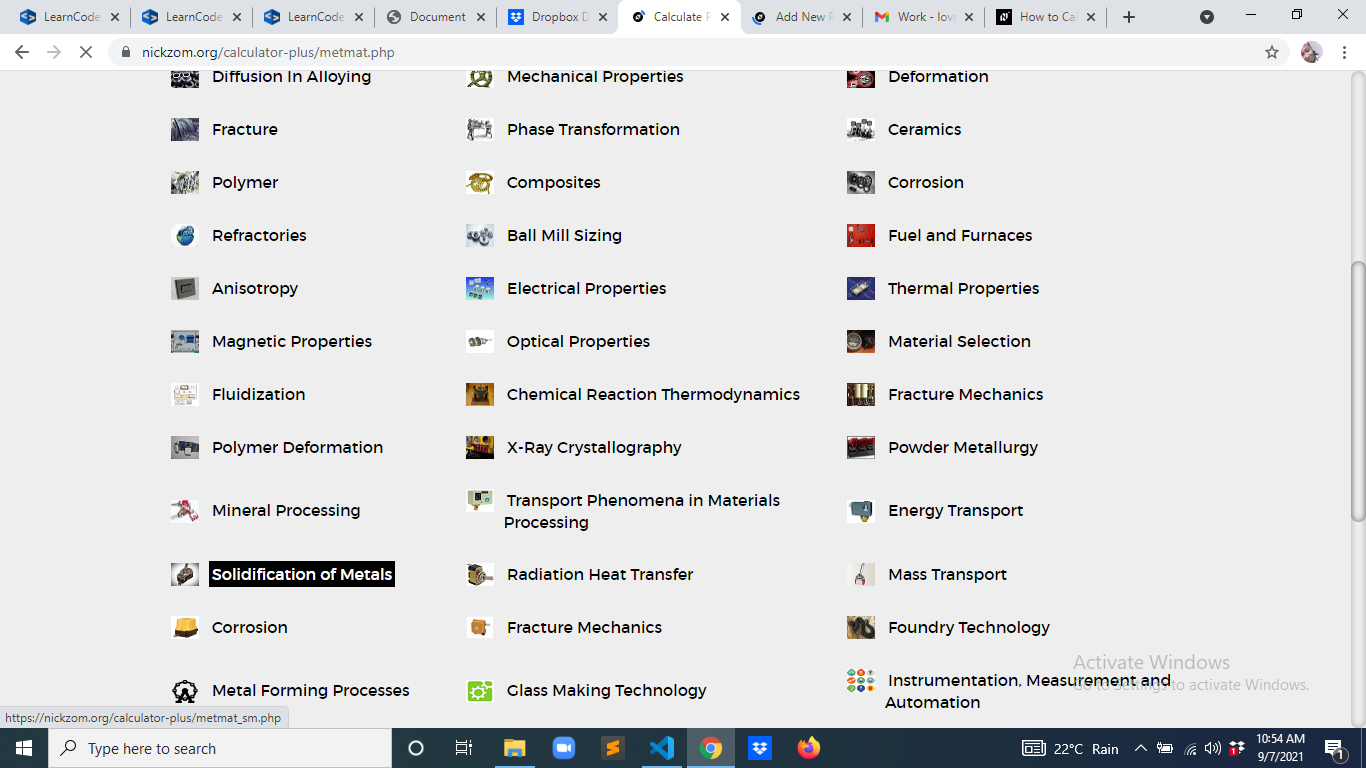Now, Click on Heat Flux under Solidification of Metal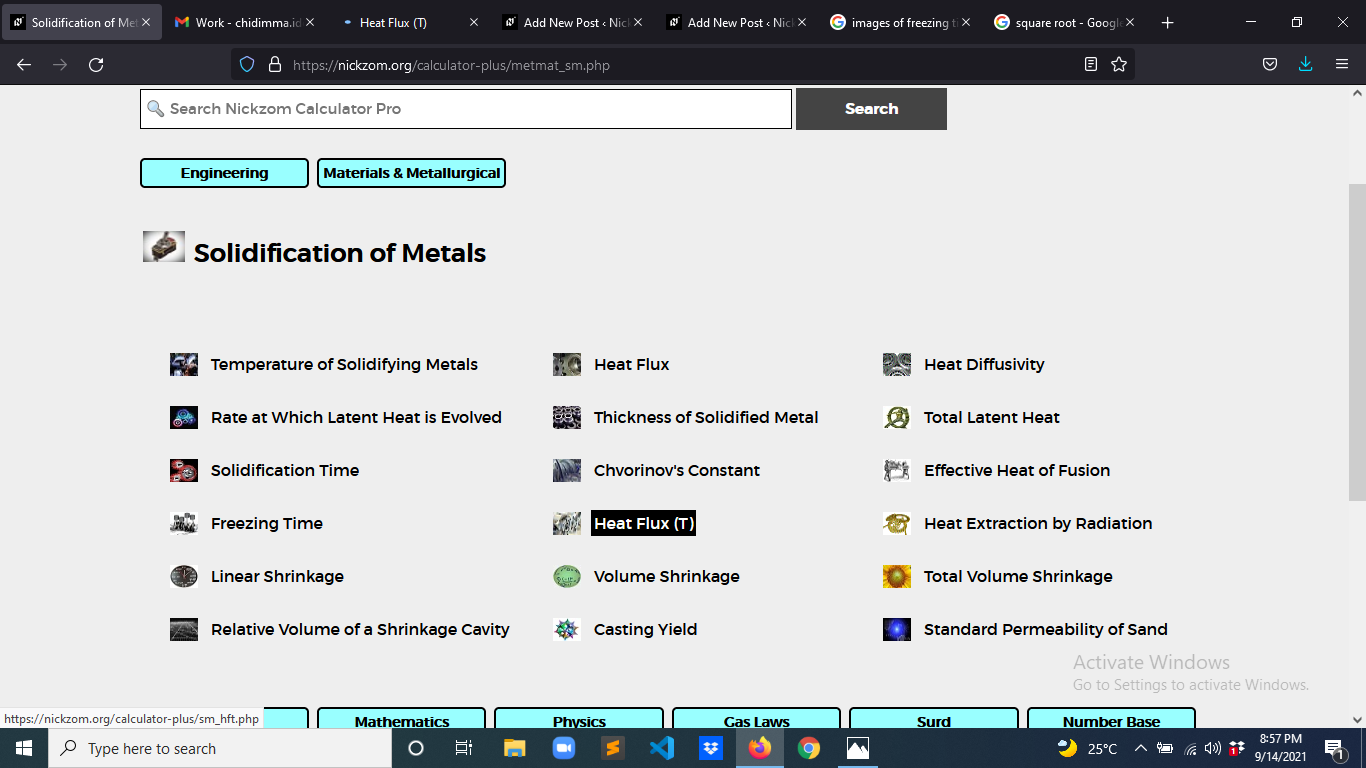The screenshot below displays the page or activity to enter your values, to get the answer for the heat flux according to the respective parameter which is the Heat Emission (h), Surface Temperature (Ts) and Initial Temperature (To).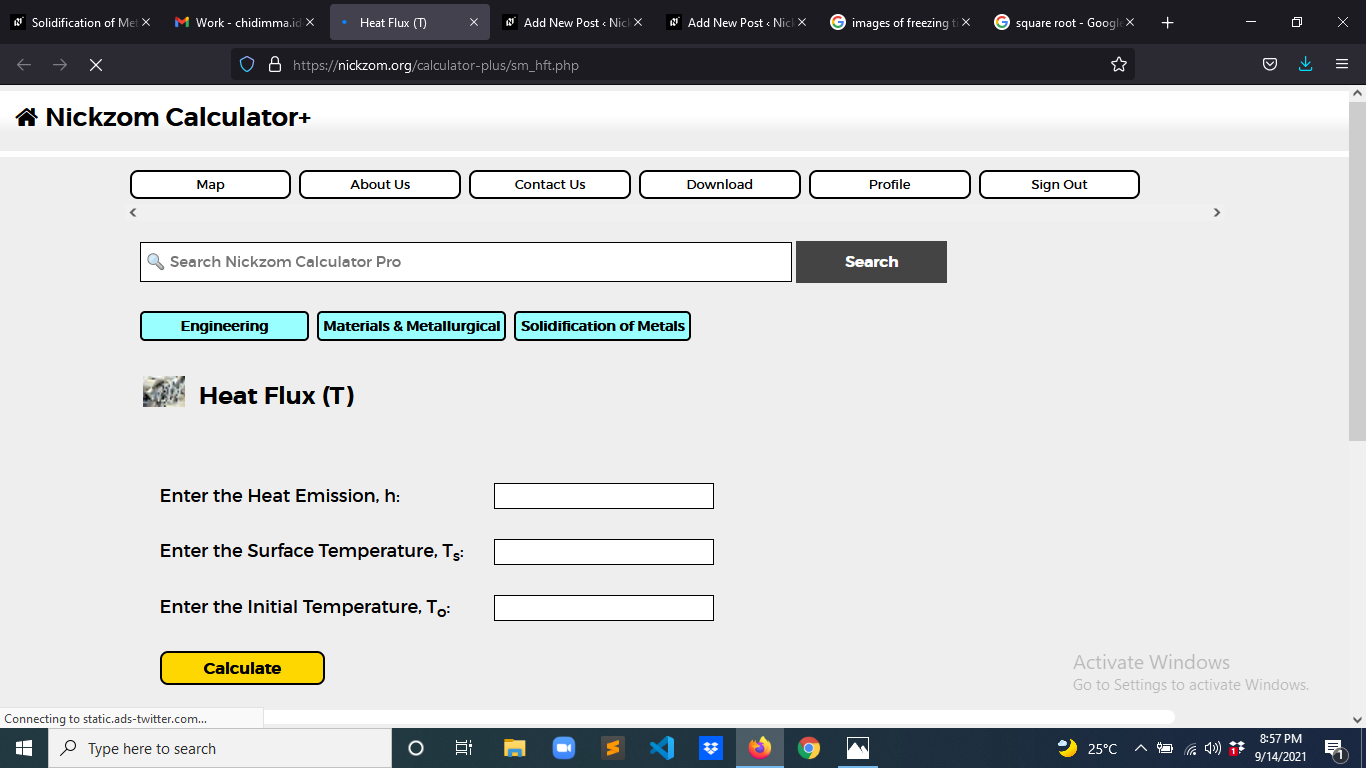Now, enter the values appropriately and accordingly for the parameters as required by the Heat Emission (h) is 21, Surface Temperature (Ts) is 12 and Initial Temperature (To) is 10.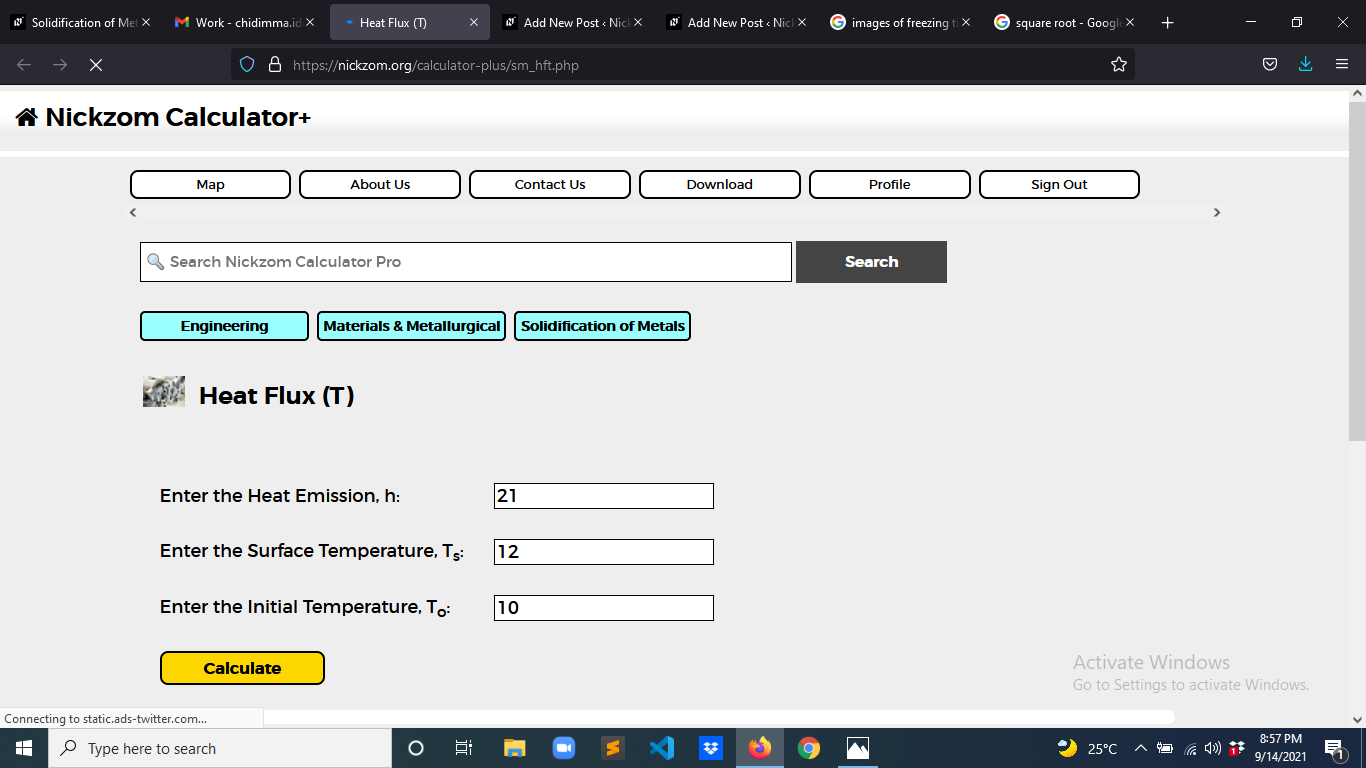Finally, Click on Calculate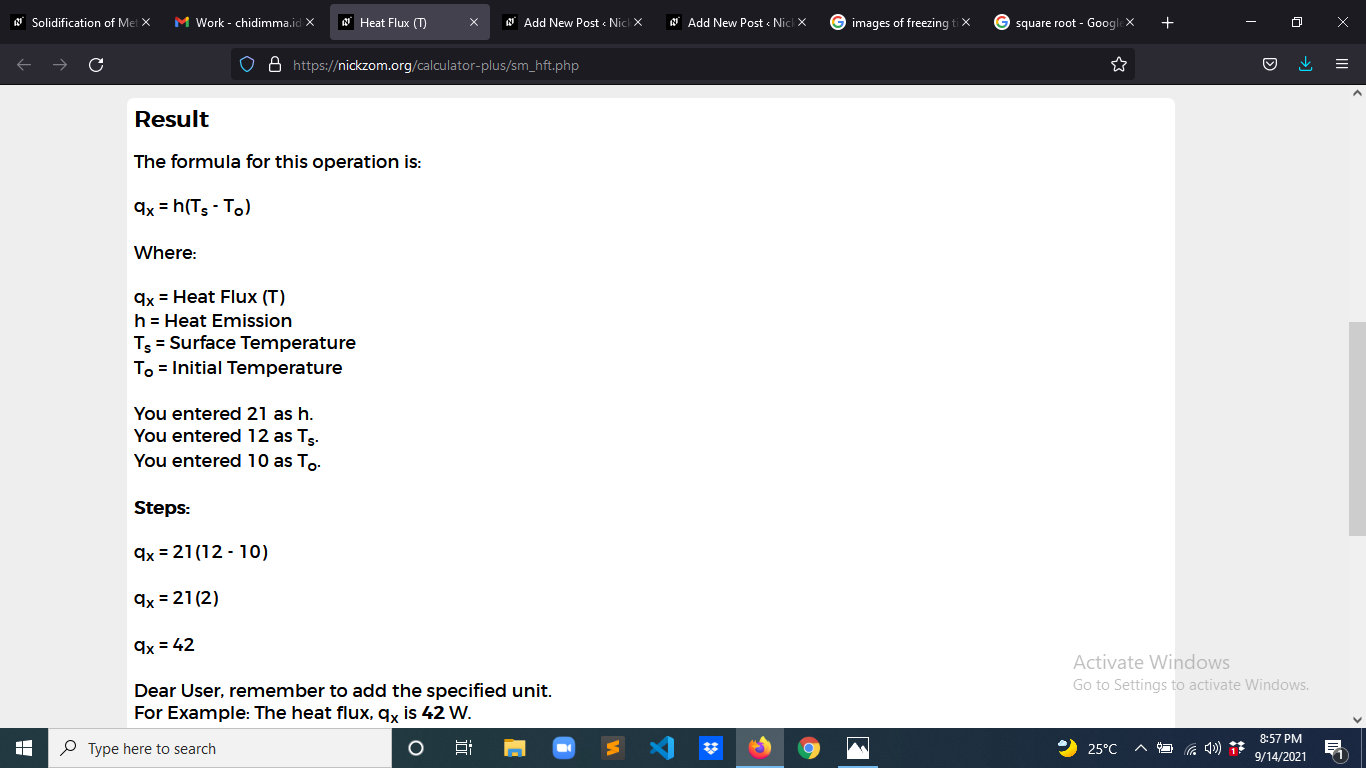As you can see from the screenshot above, Nickzom Calculator– The Calculator Encyclopedia solves for the heat flux and presents the formula, workings and steps too.